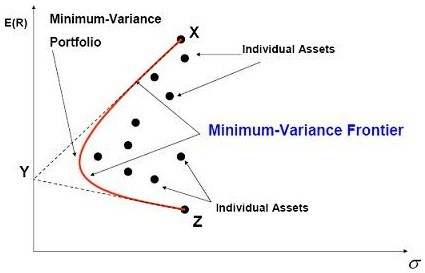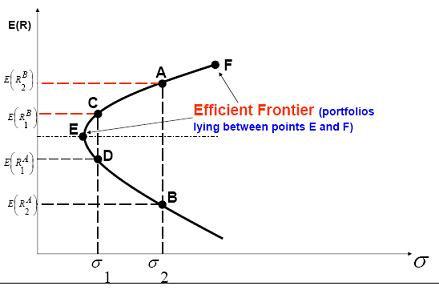### Why should I choose AnalystNotes?

AnalystNotes specializes in helping candidates pass. Period.

### Subject 2. The minimum-variance frontier

The mean-variance portfolio theory says that any investor will choose the optimal portfolio from the set of portfolios that

• Maximize expected return for a given level of risk; and
• Minimize risks for a given level of expected returns.

Again, consider a situation where you have two stocks to choose from: A and B. You can invest your entire wealth in one of these two securities. Or you can invest 10% in A and 90% in B, or 20% in A and 80%in B, or 70% in A and 30% in B, or ... There is a huge number of possible combinations and this is a simple case when considering two securities. Imagine the different combinations you have to consider when you have thousands of stocks.

The minimum variance frontier shows the minimum variance that can be achieved for a given level of expected return. To construct a minimum-variance frontier of a portfolio:

• Use historical data to estimate the mean, variance of each individual stock in the portfolio, and the correlation of each pair of stocks.
• Use a computer program to find out the weights of all stocks that minimize the portfolio variance for each pre-specified expected return.
• Calculate the expected returns and variances for all the minimum variance portfolios determined in step 2 and then graph the two variables.The outcome of risk-return combinations generated by portfolios of risky assets gives you the minimum variance for a given rate of return. Intuitively, any set of combinations formed by two risky assets with less than perfect correlation will lie inside the triangle XYZ and will be convex.

Investors will never want to hold a portfolio below the minimum variance point. They will always get higher returns along the positively sloped part of the minimum-variance frontier.

The efficient frontier is the set of mean-variance combinations from the minimum-variance frontier where for a given risk no other portfolio offers a higher expected return.Any point beneath the efficient frontier is inferior to points above. Moreover, any points along the efficient frontier, by definition, are superior to all other points for that combined risk-return tradeoff.

The portfolios on the efficient frontier have different return and risk measures. As you move upward along the efficient frontier, both risk and the expected rate of return of the portfolio increase, and no one can dominate any other on the efficient frontier. An investor will target a portfolio on the efficient frontier on the basis of his attitude toward risk and his utility curves.

The concept of efficient frontier narrows down the different portfolios from which the investor may choose. For example, portfolios at points A and B offer the same risk, but the one at point A offers a higher return for the same risk. No rational investor will hold portfolio at point B and therefore we can ignore it. In this case, A dominates B. In the same way, C dominates D.

#### Practice Question 1

Efficient portfolios are portfolios that:

I. offer the highest rate of return for the same amount of risk.
II. offer the lowest rate of return for the same amount of risk.
III. offer the lowest amount of risk for the same amount of return.
IV. offer the highest amount of risk for the same amount of return.

#### Practice Question 2

A minimum variance frontier:

I. May have at most one expected return for each level of variance.
II. May have at most two expected returns for each level of variance.
III. May have at most one variance for each level of expected return.
IV. May have at most two variances for each level of expected return.

#### Practice Question 3

Risk-averse investors may choose different efficient portfolios because:

A. some have better information than others.
B. some have higher risk tolerance than others.
C. some prefer high risk and low expected returns.

Each portfolio along the efficient frontier has a different risk-return combination, and each could have appeal to a particular investor and her degree of risk aversion.

#### Practice Question 4

Which of the following statements regarding the efficient frontier are incorrect?

I. Efficient frontier represents that set of portfolios that provides the maximum rate of return for every given level of risk.
II. Efficient frontier provides the maximum risk for each level of return.
III. Points along the efficient frontier dominate all points beneath the curve.
IV. Points along the curve to the right of any other point on the curve must have a higher expected return and higher level of risk.

The efficient frontier provides the minimum risk for each level of return. Points along the efficient frontier dominate all points beneath the curve and must have an increasing expected rate of return as they move along the curve to the right. The expected risk level also corresponds with movement to the right.

#### Practice Question 5

Stock A and B are perfectly negatively correlated. The standard deviation of A is 30% and that of B is 40%. If you construct a minimum risk portfolio with A and B, what is the proportion of stock A?

40% / (30% + 40%).

#### Practice Question 6

An efficient frontier:

I. May have at most one expected return for each level of variance.
II. May have at most two expected returns for each level of variance.
III. May have at most one variance for each level of expected return.
IV. May have at most two variances for each level of expected return.

A. I and III
B. I and IV
C. II and III

#### Practice Question 7

Set of portfolios that has the maximum rate of return for every given level of risk is called

A. the Markowitz portfolio mix.
B. the efficient frontier.
C. the minimum-variance frontier.

#### Practice Question 8

A and B are efficient portfolios. Then,

A. A combination of investments in A and B is necessarily an efficient investment.
B. If A has a higher expected return, it must have a lower risk.
C. A and B have the same risk-to-reward ratio.

An efficient frontier is made up of portfolios which have the highest expected return for a given level of risk and the lowest level of risk for a given level of expected returns. Hence, if A has a higher expected return, it must have a higher risk. However, this does not mean that A cannot have a higher risk-to-reward ratio. Finally, a combination of two efficient portfolios is always efficient (property of the frontier).

#### Practice Question 9

Which of the following is (are) true?

I. A portfolio that lies above the efficient frontier is undervalued.
II. Efficient portfolios minimize variance for a given level of expected returns.
III. A zero beta portfolio is always efficient.
IV. A stock with zero correlation coefficient with a portfolio is not useful for further diversification.

A. II only
B. I and III
C. II and IV